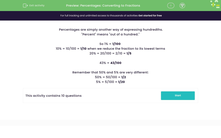# Percentages: Converting to Fractions

In this worksheet, students convert the given percentages into their fraction equivalents, giving answers in their lowest terms.Key stage:  KS 2

Curriculum topic:   Number: Fractions, Decimals and Percentages

Curriculum subtopic:   Recognise Percentages

Difficulty level:#### Worksheet Overview

Percentages are simply another way of expressing hundredths.

"Percent" means "out of a hundred."

So 1% = 1/100

10% = 10/100 = 1/10 when we reduce the fraction to its lowest terms

20% = 20/100 = 2/10 = 1/5

43% = 43/100

Remember that 50% and 5% are very different:

50% = 50/100 = 1/2

5% = 5/100 = 1/20

### What is EdPlace?

We're your National Curriculum aligned online education content provider helping each child succeed in English, maths and science from year 1 to GCSE. With an EdPlace account you’ll be able to track and measure progress, helping each child achieve their best. We build confidence and attainment by personalising each child’s learning at a level that suits them.

Get started